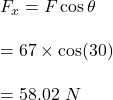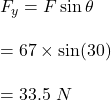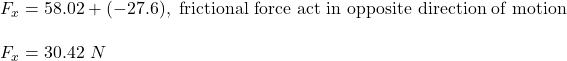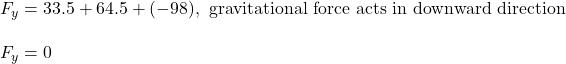## At one moment during a walk around the block, there are four forces exerted upon Fido – a 10.0 kg dog. The forces are: Fapp = 67

Question

At one moment during a walk around the block, there are four forces exerted upon Fido – a 10.0 kg dog. The forces are:

Fapp = 67.0 N at 30.0° above the horizontal (rightward and upward)
Fnorm = 64.5 N, up
Ffrict = 27.6 N, left
Fgrav = 98 N, down

Resolve the applied force (Fapp) into horizontal and vertical components. Then add the forces up as vectors to determine the net force.

in progress 0
6 months 2021-07-17T08:02:56+00:00 1 Answers 73 views 0

1. Explanation:

Given that,

Fapp = 67.0 N at 30.0° above the horizontal (rightward and upward)

Fnorm = 64.5 N, up

Ffrict = 27.6 N, left

Fgrav = 98 N, down

(a) Resolution of the applied force:

Horizontal component,Vertical component,(b) Net horizontal force :It is positive, it will act in right side

Net vertical force :Hence, it is clear that the net force is in horizontal direction i.e. 30.42 N due right side.